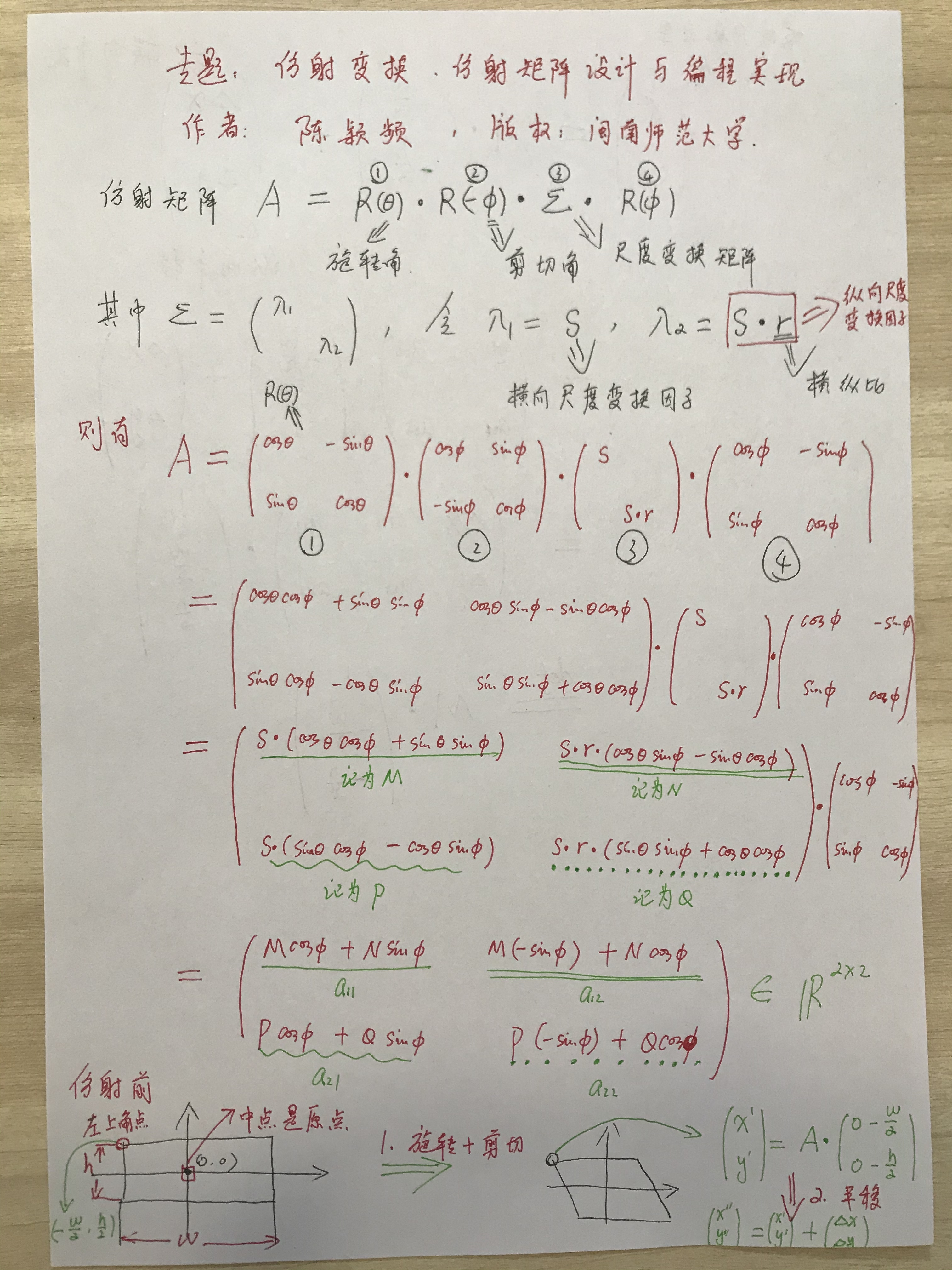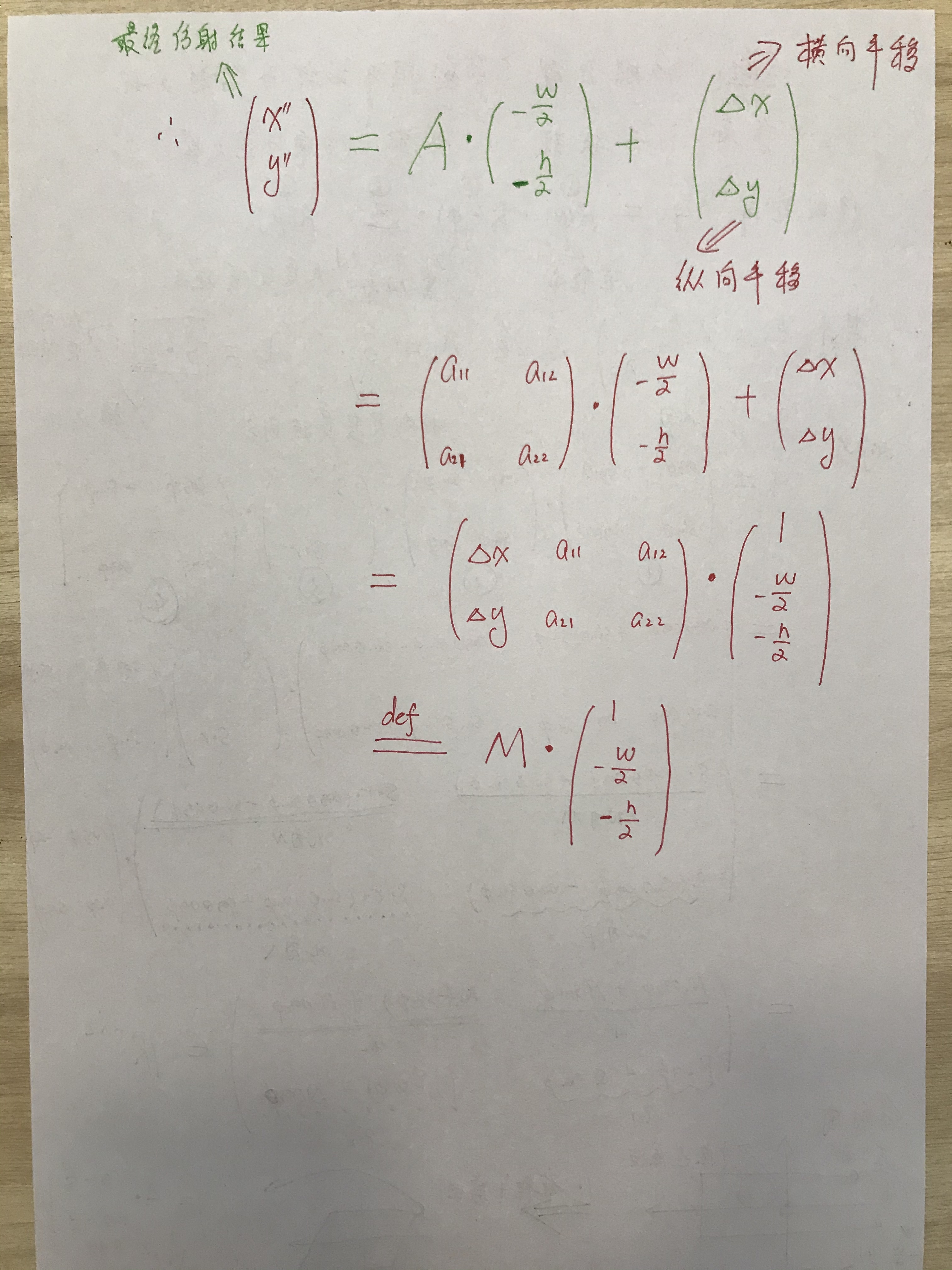# IVT目标跟踪中的仿射变换矩阵设计详解与编程实现

笔者近期研究目标跟踪，涉及到运动表观模型的动态建模，需要进行仿射变换。我阅读了L1-APG和IVT算法、ALSA算法、SCM算法中的仿射变换，发现这几种算法的仿射变换实现代码有非常大的差异。其中IVT算法的仿射变换似乎在各种视觉跟踪算法中用得更多，但是代码上没有做很详细的解释，我查找各类原创的论文、博客，似乎也没有讲清楚IVT仿射变换代码实现的基本原理。IVT中用到affparam2mat.m这个函数进行仿射矩阵的编程，但是我发现这个代码和一般的仿射变换公式推导对不上号，于是我自己试图去揣测作者的想法，完整地重建出这个作者仿射变换矩阵的设计过程。IVT这个仿射变换的关键在于，第一：它假定仿射变换之前，那个矩形的中心是在原点的，第二：IVT的仿射是两步完成的，先做旋转切变，然后再做平移；第三：IVT仿射变换中的A矩阵，是采取奇异值分解的方式进行的，需要用到旋转角，切变角，尺度变换因子，高宽比这四个随机变量。因此，这个体系就和L1-APG完全不同，具体设计细节如下：function q = affparam2mat(p)% function q = affparam2mat(p)%%   input  :  p， a 'geometric' affine parameter;%   output  ： q， a 2x3 matrix;%% The functions affparam2geom and affparam2mat convert a 'geometric'% affine parameter from/to a matrix form (2x3 matrix).%% affparam2mat converts 6 affine parameters (x, y, th, scale, aspect, skew) to a 2x3 matrix, % and affparam2geom does the inverse.%%    p(6) : [dx dy sc th sr phi]'%    q(6) : [q(1) q(3) q(4); q(2) q(5) q(6)]%% Reference "Multiple View Geometry in Computer Vision" by Richard% Hartley and Andrew Zisserman. % Copyright (C) Jongwoo Lim and David Ross.  All rights reserved.% Thanks to Jongwoo Lim and David Ross for this code.  -- Wei Zhong.sz = size(p);if (length(p(:)) == 6)  p = p(:);ends = p(3,:);  th = p(4,:);  r = p(5,:);  phi = p(6,:);cth = cos(th);  sth = sin(th);  cph = cos(phi);  sph = sin(phi);ccc = cth.*cph.*cph;  ccs = cth.*cph.*sph;  css = cth.*sph.*sph;scc = sth.*cph.*cph;  scs = sth.*cph.*sph;  sss = sth.*sph.*sph;q(1,:) = p(1,:);  q(2,:) = p(2,:);q(3,:) = s.*(ccc +scs +r.*(css -scs));  q(4,:) = s.*(r.*(ccs -scc) -ccs -sss);q(5,:) = s.*(scc -ccs +r.*(ccs +sss));  q(6,:) = s.*(r.*(ccc +scs) -scs +css);q = reshape(q, sz);

闽南师范大学 陈颖频

https://blog.sciencenet.cn/blog-684084-1231326.html

## 全部精选博文导读

GMT+8, 2021-12-6 20:33